## RS Aggarwal Class 7 Solutions Chapter 6 Algebraic Expressions Ex 6B

These Solutions are part of RS Aggarwal Solutions Class 7. Here we have given RS Aggarwal Solutions Class 7 Chapter 6 Algebraic Expressions Ex 6B.

Other Exercises

Find the products:
Question 1.
Solution:
3 x 8 a2+4 = 24a6

Question 2.
Solution:
(-6x3) x (5x2) = -6 x 5x2+3 = -30x5

Question 3.
Solution:
(-4ab) x (-3a2bc)
= (-4) x (-3) a. a2.b. b. c
= 12.a2+1. b1+1.c
= 12a3b2c

Question 4.
Solution:
= (2a2b3) x (-3a3b)
= 2 x (-3) a2. a3. b3. b. b
= -6a2+3.b3+1
= -6a5.b4

Question 5.
Solution: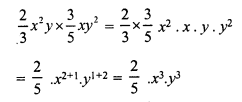Question 6.
Solution: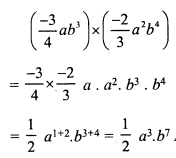Question 7.
Solution:Question 8.
Solution:Question 9.
Solution:Question 10.
Solution:Question 11.
Solution: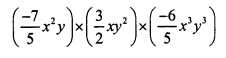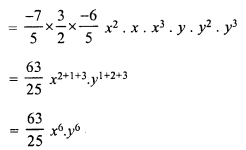Question 12.
Solution:Question 13.
Solution: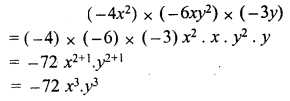Question 14.
Solution:Question 15.
Solution: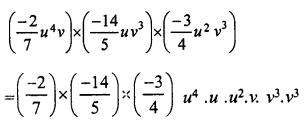Question 16.
Solution:Question 17.
Solution: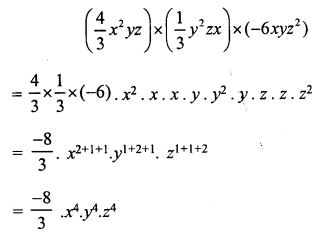Question 18.
Solution: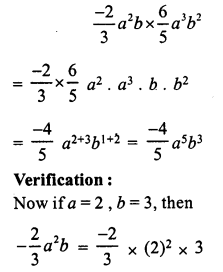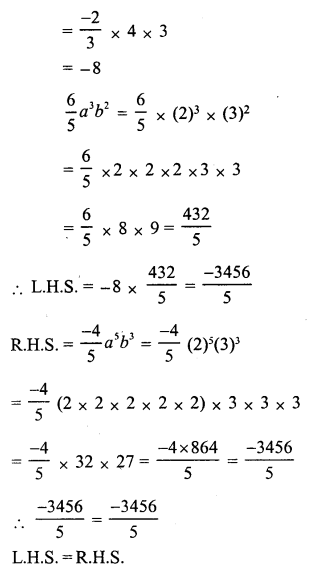Question 19.
Solution:Question 20.
Solution: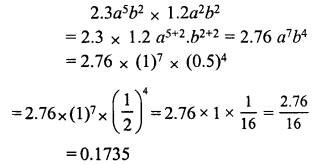Question 21.
Solution:Find the products given below and in each case verify the result for a = 1, b = 2 and c = 3 :
Question 22.
Solution: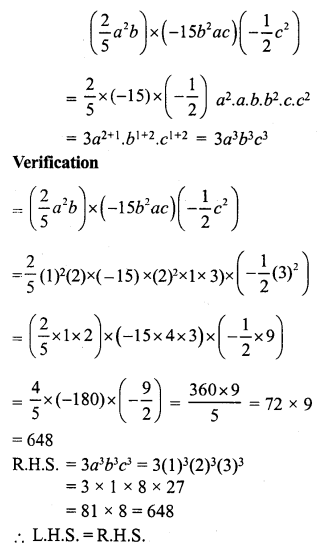Question 23.
Solution: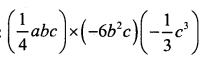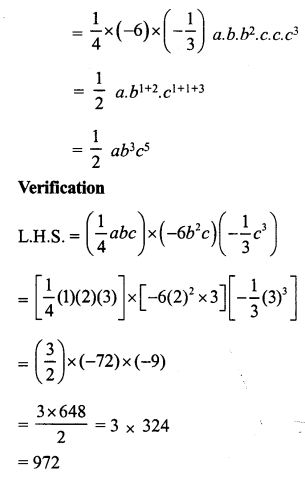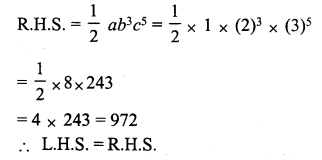Question 24.
Solution: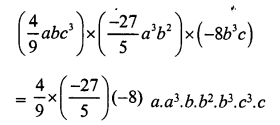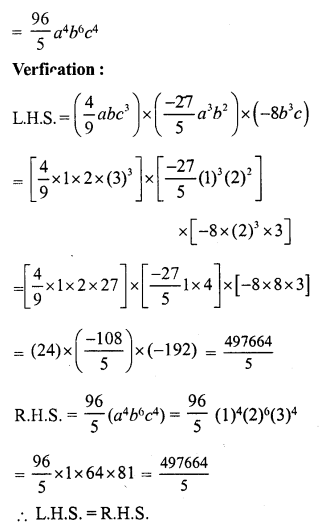Question 25.
Solution:Hope given RS Aggarwal Solutions Class 7 Chapter 6 Algebraic Expressions Ex 6B are helpful to complete your math homework.

If you have any doubts, please comment below. Learn Insta try to provide online math tutoring for you.